SYLLABUS  Previous: 1.4 Numerical discretization  Up: 1.4 Numerical discretization  Next: 1.4.2 Sampling on a

### 1.4.1 Convergence, Richardson extrapolation

One of the most important properties of a numerical approximation based on a finite number of data points is that the approximation converges to the exact value as more information is gathered (exercise 1.01). This convergence can be defined locally for any arbitrary point in space and time (more restrictive) or by monitoring a global quantity (more permissive).

The convergence rate can be estimated experimentally from a geometric sequence of approximations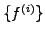, by successively refining the numerical resolution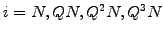, for example by doubling the resolution a couple of times when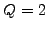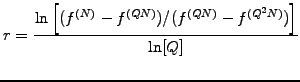(1.4.1#eq.1)

Knowing the convergence rate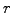, it is possible to further improve the accuracy of the numerical approximation by using a so-called Richardson extrapolation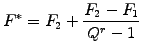(1.4.1#eq.2)

where a more accurate solution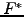is obtained from an evaluation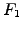and its refinement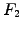.

Let's take an example and approximate the function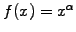on a grid in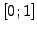, simply by sampling the value of the function in the middle of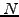intervals at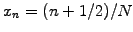. The approximation for the value at the origin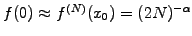converges to zero provided that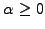, which is best visualized in a convergence study using a lin-lin plot (figure 1.4.1#fig.1, left).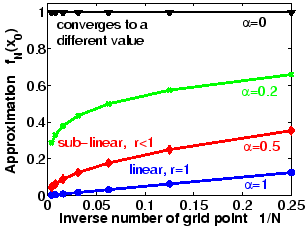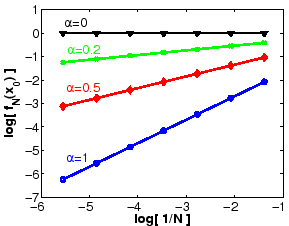The convergence rate for the approximation measured at the origin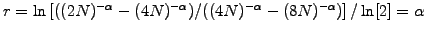drops as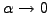as shown by the lower slope in the log-log plot (figure 1.4.1#fig.1, right). Not apparent in the log-log plot is that the approximation converges to a different value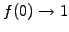when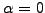.

The mid-point rule (sect.3.3) can be used to approximate an integral by computing the area of small boxes in order to evaluate the global quantity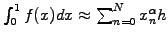with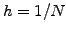. Global convergence is achieved for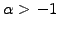; because of the weak singularity, the convergence rate drops from the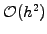expected from a quadrature of smooth functions to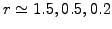when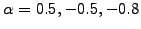.

SYLLABUS  Previous: 1.4 Numerical discretization  Up: 1.4 Numerical discretization  Next: 1.4.2 Sampling on a